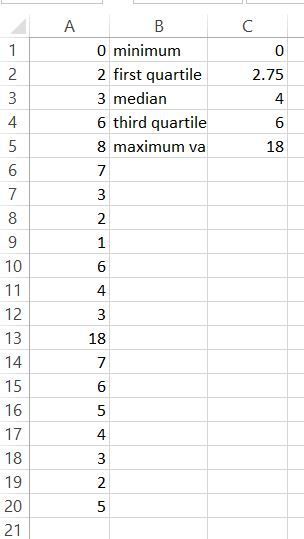#### How to Calculate Quartiles

Quartiles are used to divide a set of data into four equal parts. In this Excel tutorial, you will teach yourself how to calculate quartiles.

## Use of the QUARTILE.INC function

To calculate quartiles in Excel just use QUARTILE.INC function. The QUARTILE function in Excel returns the quartile of a set of data.

QUARTILE.INC syntax is:

=QUARTILE.INC(array, quart)

where:

quart is a number of quartile which you want to calculate

Example:

Your data table is in A1:A10 range and you want to calculate second quartile.

## How to calculate a second quartile?

To calculate second quartile just use this function:

=IFERROR(QUARTILE.INC(A1:A20,2),”No Values”)

IFERROR function in this formula prevents you from #NUM! error. It happens when your data table will be empty.

## Examples of calculating quartiles

You can set quart in QUARTILE.INC formula to 0 – 4.

=IFERROR(QUARTILE.INC(A1:A20;0);”No Values”) – shows minimum value

=IFERROR(QUARTILE.INC(A1:A20,1),”No Values”) – calculates first quartile

=IFERROR(QUARTILE.INC(A1:A20,2),”No Values”) – calculates second quartile (which is median)

=IFERROR(QUARTILE.INC(A1:A20,3),”No Values”) – calculates third quartile

=IFERROR(QUARTILE.INC(A1:A20,4),”No Values”) – shows maximum value## Use of the PERCENTILE function

• The PERCENTILE function in Excel returns the nth percentile of a set of data. The syntax for the PERCENTILE function is as follows: =PERCENTILE(array, k).
• “Array” is the range of cells that contains the data.
• “K” is the percentile that you want to calculate. It is a number between 0 and 1, inclusive.
• For example, to calculate the first quartile (25th percentile), set k to 0.25. To calculate the second quartile (50th percentile), set k to 0.5, and so on.
• For example, if your data is in the range A1:A10, to calculate the first quartile, enter the formula =PERCENTILE(A1:A10,0.25) in a cell.